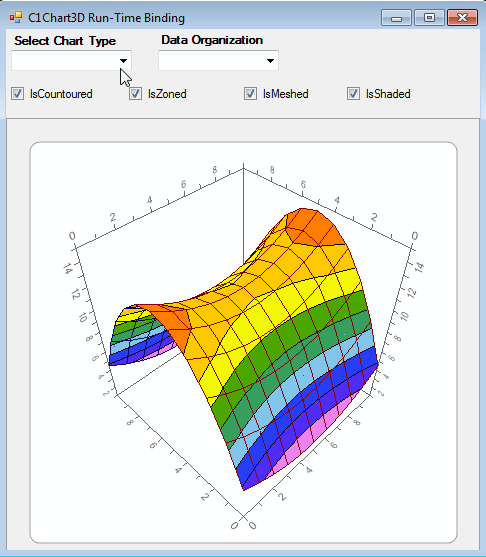﻿ Runtime Binding in C1Chart3D# Runtime Binding in C1Chart3D

Displaying Chart in a 3D view is not only discerning but exciting too, and the run time interaction and clear view of the data proves to be a plus point to its features.In this blog, we will be discussing the simple ways of binding data at run-time. C1Chart3D allows you to display three types of charts: Bar, Scatter and Surface. And with these different types are the different ways of binding:

1. Regular Grid DataSet
2. Irregular Grid DataSet
3. Point DataSet

## Regular Grid DataSet

Grid layouts are used when the X-coordinates of each point and the Y-coordinates of each point are always the same distance apart. To bind C1Chart3D with a Regular DataSet, you would need to create a Chart3DDataSetGrid object and then set it for C1Chart3D. Below is the code that can be used for the purpose:

``````
//Setting Regular Grid Data
int i, j;
double[,] z = new double[21, 21];
for (i = 0; i < z.GetLength(0); i++)
{
for (j = 0; j < z.GetLength(1); j++)
{
z[i,j] = 200 - ((i - 10) * (i - 10) + (j - 10) * (j - 10));
}
}
Chart3DDataSetGrid gridset = new Chart3DDataSetGrid(0, 0, 1, 1, z);
c1Chart3D1.ChartGroups.ChartData.SetGrid = gridset;

``````

## Irregular Grid DataSet

Irregular Grid layouts are used when the X-coordinates of each point or the Y-coordinates of each point are not the same distance apart. To bind C1Chart3D with a Irregular DataSet, you would need to create a Chart3DDataSetIrGrid object and then set it for C1Chart3D.

``````
//Setting Irregular Grid Data
int i, j;
double[] x = new double;
double[] y = new double; ;
double[,] z = new double[21, 21];
for (i = 0; i < 21; i++)
{
x[i] = i * 07.122;
y[i] = i * 04.562;
}
for (i = 0; i < 21; i++)
{
for (j = 0; j < 21; j++)
{
z[i,j] = ((i - 10.2) * (i + 17.2) + (j + 10.2) * (j - 1.2));
}

}
Chart3DDataSetIrGrid irrgrieset = new Chart3DDataSetIrGrid(x, y, z);
c1Chart3D1.ChartGroups.ChartData.SetIrGrid = irrgrieset;

``````

## Point DataSet

The Point Data Layout is used only for scatter plots when charting multiple series of points. Below is the sample code to bind a chart with Point Data:

``````
//Setting Point data
Chart3DDataSetPoint setPoint = new Chart3DDataSetPoint();
Chart3DPoint[] pointArr = new Chart3DPoint;
Chart3DPoint point;
for (int i = 0; i < 50; i++)
{
if (i % 2 == 0)
{
point = new Chart3DPoint(i * 1.1, i * 72, i * 3);
pointArr[i] = point;
}
else
{
point = new Chart3DPoint(i * 0.1, i * 0.3, i * 7.4);
pointArr[i] = point;
}
}
for (int i = 50; i < 100; i++)
{
if (i % 2 == 0)
{
point = new Chart3DPoint(i * 5.5, i * 6.1, i * 0.003);
pointArr[i] = point;
}
else
{
point = new Chart3DPoint(i * 0.1, i * 0.3, i * 7.4);
pointArr[i] = point;
}
}